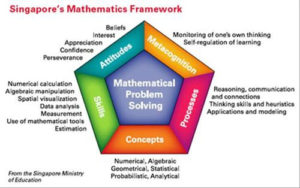# MathematicsWe believe that all mathematical learning is an opportunity for developing critical thinking and problem solving skills using multiple strategies. In addition, we emphasize real-world applications of mathematical ideas so that students are able to connect and apply the math skills learned at JCDSRI to the world they live in and to understand the importance of math in their lives. An important consideration of our math program is that we meet the needs of all students to satisfy their various learning styles by using different teaching modalities.

We use the Math in Focus curriculum. Math in Focus is based on the Singapore approach. It focuses on problem solving as the foundation of mathematical learning and uses a variety of models to help students visualize and understand mathematical concepts. This program teaches concepts using a concrete-pictorial-abstract learning progression to anchor student knowledge in real-world, hands-on experiences supported by technology applications. Math in Focus encourages positive student interaction with mathematics which results in students developing an ability to easily solve complex, real-world math problems.

The math curriculum that gives all grade levels an opportunity to deepen their understanding of essential math skills such as number concepts, geometric principles, financial literacy, patterning, measurement, and data analysis. At the same time we work toward proficiency in number sense and computation skills.